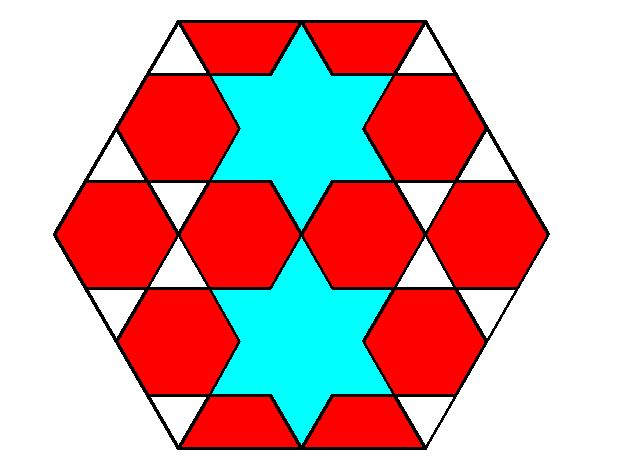# Cool shapes

Geometry Level 2Given that the area of the big hexagon is $23.38 \text{cm}^2$, what is the area of the blue region? (Give the answer in $\text{cm}^2$ up to two decimal places)

×